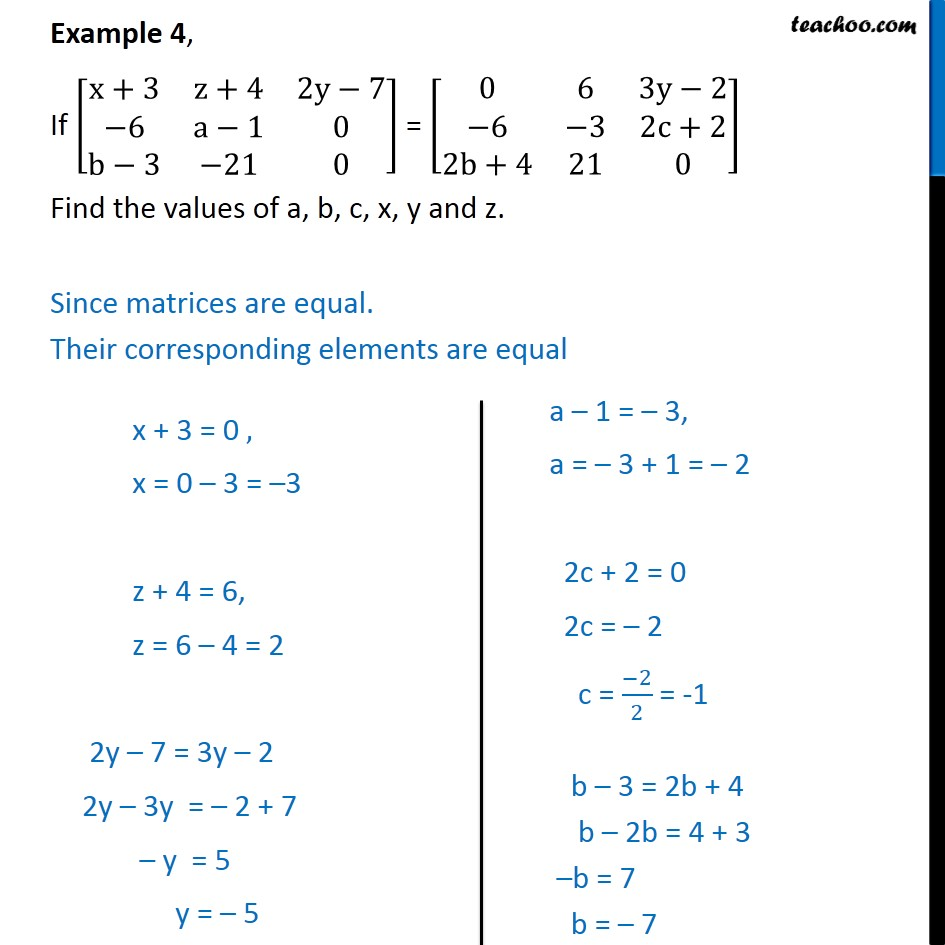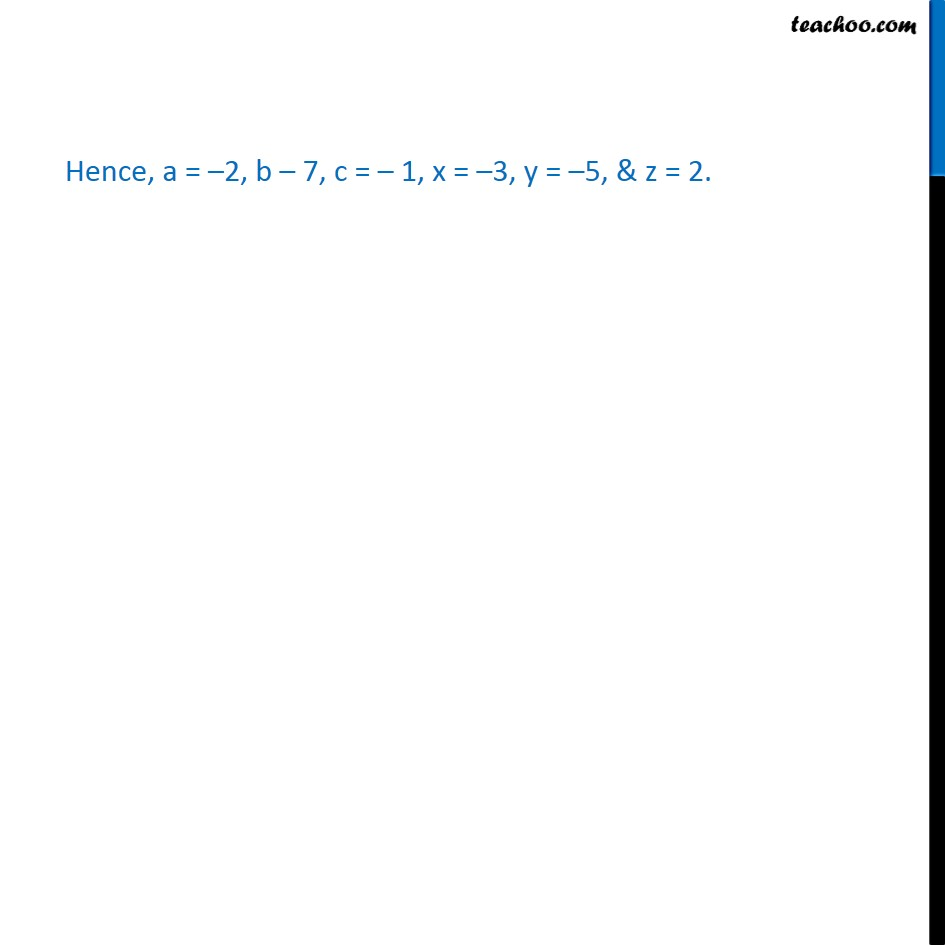Learn All Concepts of Chapter 3 Class 12 Matrices - FREE. Check - Matrices Class 12 - Full video1. Chapter 3 Class 12 Matrices
2. Concept wise
3. Equal matrices

Transcript

Example 4, If [ 8(x+3&z+4&2y 7@ 6&a 1&0@b 3& 21&0)] = [ 8(0&6&3y 2@ 6& 3&2c+2@2b+4&21&0)] Find the values of a, b, c, x, y and z. Since matrices are equal. Their corresponding elements are equal Hence, a = 2, b 7, c = 1, x = 3, y = 5, & z = 2.

Equal matrices